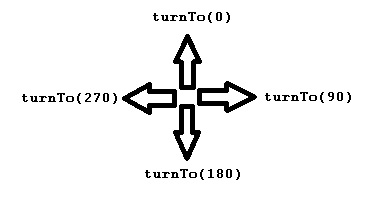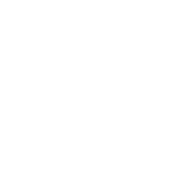# turnTo

Category:Turtle

## turnTo(angle)

Category: Turtle

Changes the turtle's direction to a specific angle. 0 is up, 90 is right, 180 is down, and 270 is left.

Sometimes you need to orient the turtle in a certain direction before drawing something. turnTo changes the turtle's direction to be angle degrees clockwise from a line pointing up. The turtle's position remains the same.### Examples

```// Turtle faces right.
turnTo(90);
```

Example: Look South Turtle faces down.

```// Turtle faces down.
turnTo(180);
```

Example: Clock Face Draw a minute and hour marks on a clock face

 ```// Draw a minute and five minute marks on a clock face for (var minute = 0; minute <= 60; minute++) { penUp(); moveTo(160, 240); turnTo(minute*6); // Every minute is 6 degrees. penUp(); if(Math.round(minute/5)*5==minute) { // Must be a five minute mark. moveForward(50); penDown(); moveForward(25); } else { moveForward(70); penDown(); moveForward(5); } } ```### Syntax

```turnTo(angle);
```

### Parameters

Name Type Required? Description
angle number Yes The angle to point the turtle.

### Returns

No return value. Rotates turtle only.

### Tips

• You can specify a negative angle to rotate to, measured counterclockwise from up (0 angle).
• There are three ways to rotate the turtle in place
• turnRight(angle) - Rotates the turtle right by the specified angle relative to the current turtle direction. The turtle’s position remains the same.
• turnLeft(angle) - Rotates the turtle left by the specified angle relative to the current turtle direction. The turtle’s position remains the same.
• turnTo(angle) - Rotates the turtle to a specific angle. 0 is up, 90 is right, 180 is down, and 270 is left. The turtle’s position remains the same.

Found a bug in the documentation? Let us know at documentation@code.org

Found a bug in the documentation? Let us know at documentation@code.org